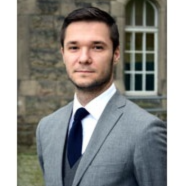# Jakub Ciesielski - Science profile - MOST Wiedzy

## Search## mgr inż. Jakub Ciesielski

### Assistant

Workplace
Gmach B
pokój 503 open in new tab
Phone
58 347 10 09
E-mail
jakub.ciesielski@pg.edu.pl

### Publication showcase

• #### Bernstein-type theorem for ϕ-Laplacian

In this paper we obtain a solution to the second-order boundary value problem of the form \frac{d}{dt}\varPhi'(\dot{u})=f(t,u,\dot{u}), t\in [0,1], u\colon \mathbb {R}\to \mathbb {R} with Sturm–Liouville boundary conditions, where \varPhi\colon \mathbb {R}\to \mathbb {R} is a strictly convex, differentiable function and f\colon[0,1]\times \mathbb {R}\times \mathbb {R}\to \mathbb {R} is continuous and satisfies a suitable growth...

Full text available

• #### On the Existence of Homoclinic Type Solutions of a Class of Inhomogenous Second Order Hamiltonian Systems

We show the existence of homoclinic type solutions of a class of inhomogenous second order Hamiltonian systems, where a C1-smooth potential satisfies a relaxed superquadratic growth condition, its gradient is bounded in the time variable, and a forcing term is sufficiently small in the space of square integrable functions. The idea of our proof is to approximate the original system by time-periodic ones, with larger and larger...

Full text available

• #### On the existence of homoclinic type solutions of inhomogenous Lagrangian systems

We study the existence of homoclinic type solutions for a class of inhomogenous Lagrangian systems with a potential satisfying the Ambrosetti-Rabinowitz superquadratic growth condition and a square integrable forcing term. A homoclinic type solution is obtained as a limit of periodic solutions of an approximative sequence of second order differential equations.

Full text in external service

seen 584 times